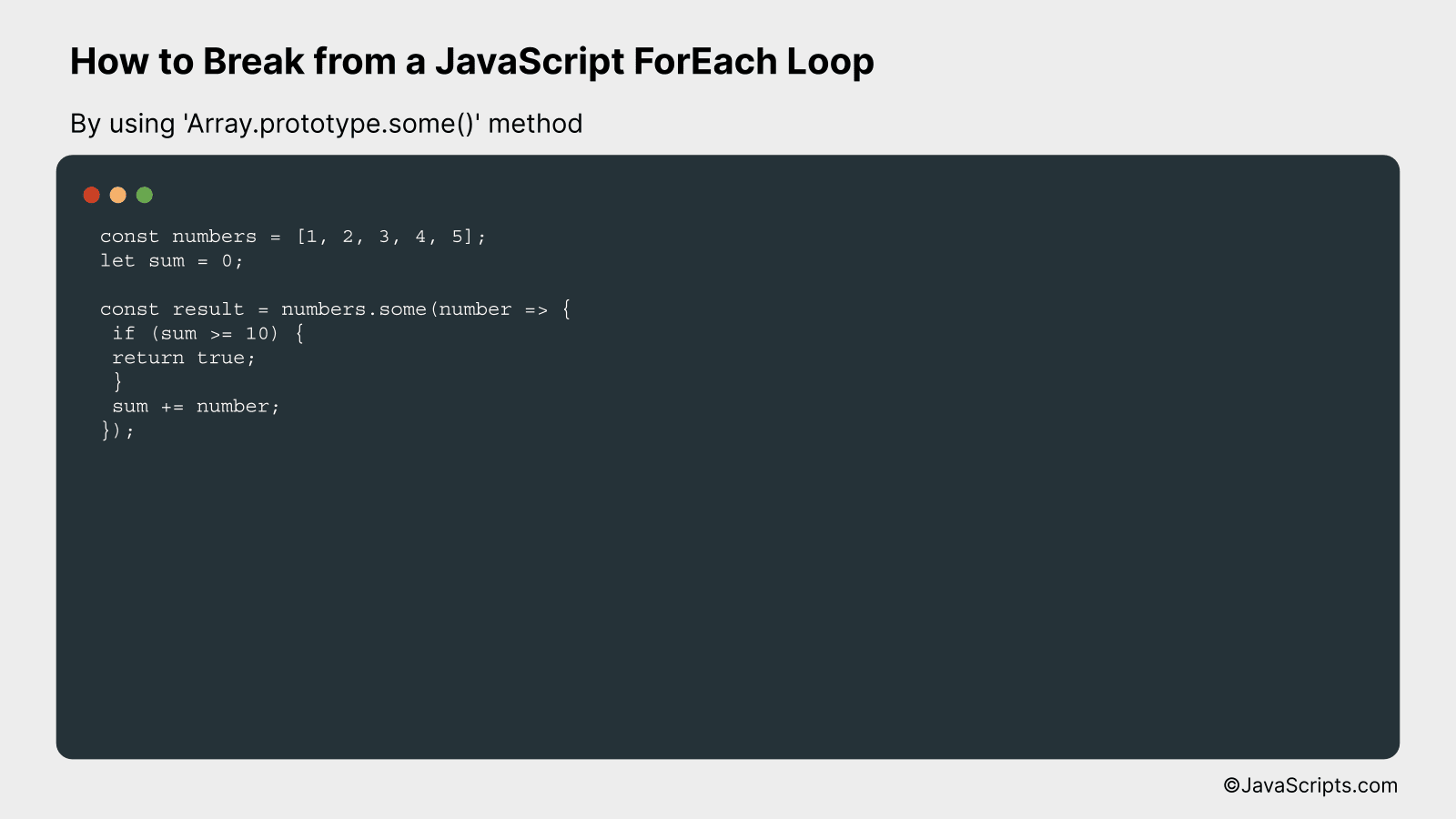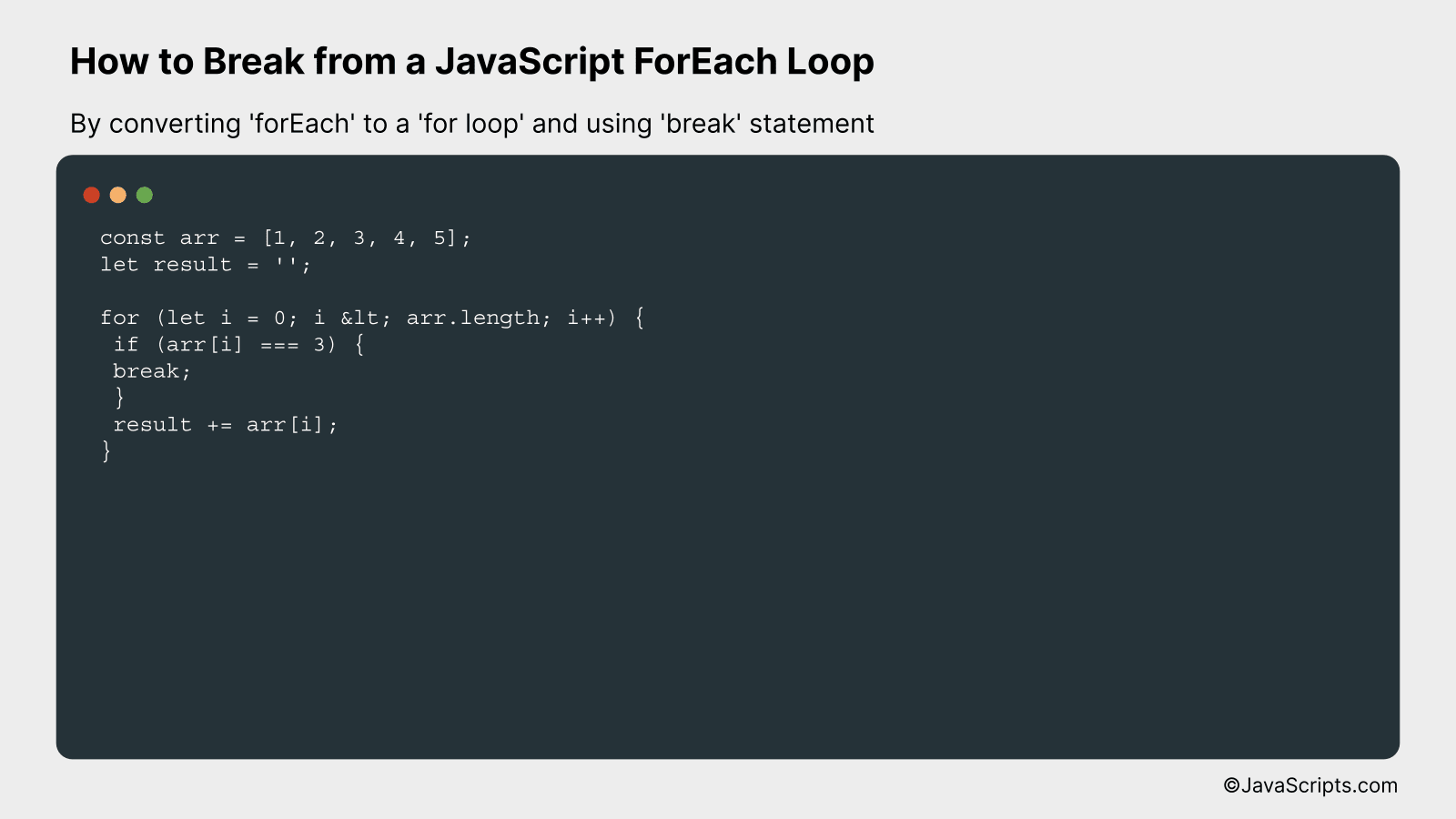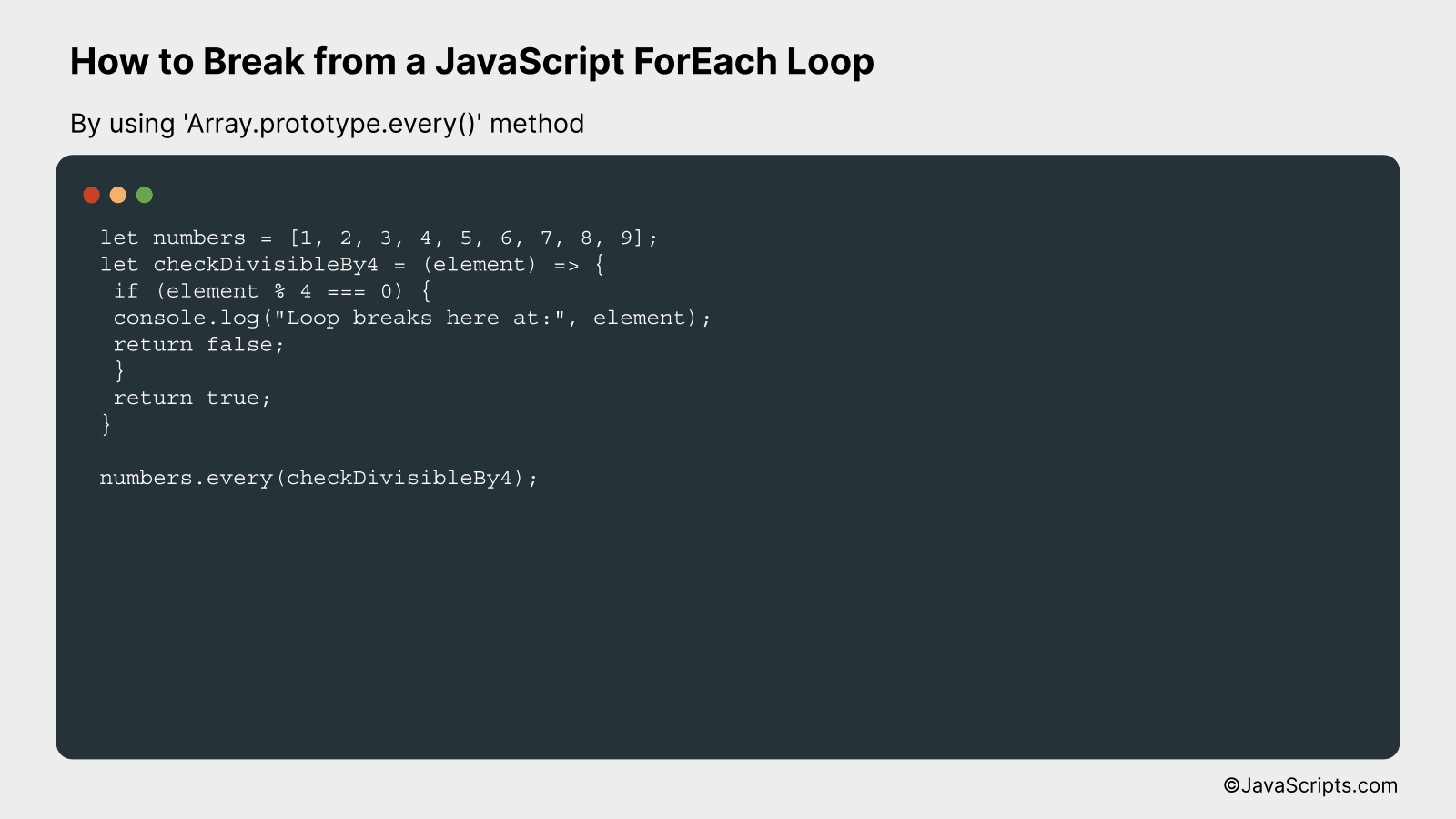# How to Break from a JavaScript ForEach Loop

Breaking free from a JavaScript ForEach loop can seem a bit daunting if you’re new to the JavaScript world or if you’re unsure about how to maneuver through loops efficiently. This is because, unlike traditional ‘for’ or ‘while’ loops, a ForEach loop doesn’t offer a straightforward mechanism to break out of the loop.

So how do we overcome this challenge? Let’s make it simple. We’ll walk through some effective and easy-to-understand strategies to break out of a ForEach loop. Together, we’ll demystify this concept, equipping you with one more JavaScript tool to use confidently in your coding adventures.

In this post, we will be looking at the following 3 ways to break from a JavaScript forEach loop:

• By using ‘Array.prototype.some()’ method
• By converting ‘forEach’ to a ‘for loop’ and using ‘break’ statement
• By using ‘Array.prototype.every()’ method

Let’s explore each…

## #1 – By using ‘Array.prototype.some()’ methodUsing ‘Array.prototype.some()’ method, you can break from the loop once a condition is met. It works by iterating over the array until the provided function returns ‘true’. Here’s an example:

``````
const numbers = [1, 2, 3, 4, 5];
let sum = 0;

const result = numbers.some(number => {
if (sum >= 10) {
return true;
}
sum += number;
});
``````

### How it works

The ‘Array.prototype.some()’ method is used to test whether at least one element in the array passes the test implemented by the provided function, and it stops iterating once it finds a ‘true’ match.

• Declare a ‘numbers’ array containing integers.
• Initialize a ‘sum’ variable to 0.
• Call the ‘some()’ method on the ‘numbers’ array, providing it a function that will be executed for each element in the array until the function returns ‘true’ or all elements have been processed.
• In the provided function, check if ‘sum’ is greater than or equal to 10, if so, return ‘true’ to break out of the loop.
• If the condition is not met, add the current ‘number’ to the ‘sum’ variable.
• Once the loop is broken or finished iterating, the result variable will store whether the condition was met or not.

## #2 – By converting ‘forEach’ to a ‘for loop’ and using ‘break’ statementConvert a JavaScript forEach loop to a for loop to enable using the break statement, as demonstrated in the following example:

``````
const arr = [1, 2, 3, 4, 5];
let result = '';

for (let i = 0; i < arr.length; i++) {
if (arr[i] === 3) {
break;
}
result += arr[i];
}
``````

### How it works

This conversion allows you to use the 'break' statement within the loop, effectively stopping the loop when a specific condition is met. Here's a step-by-step explanation:

• Define an array `arr` and a variable `result` to store the concatenated elements.
• Replace the forEach loop with a regular `for` loop that iterates through the array.
• Inside the for loop, check if the current element is equal to 3.
• If the element is 3, use the `break` statement to exit the loop.
• If the element is not 3, concatenate it to the `result` variable.

## #3 - By using 'Array.prototype.every()' methodThe 'Array.prototype.every()' method in JavaScript is a viable way to break from a loop early. It iterates over an array and stops once it encounters a falsy return from the provided function, effectively simulating a 'break' in a traditional loop.

``````
let numbers = [1, 2, 3, 4, 5, 6, 7, 8, 9];
let checkDivisibleBy4 = (element) => {
if (element % 4 === 0) {
console.log("Loop breaks here at:", element);
return false;
}
return true;
}

numbers.every(checkDivisibleBy4);
``````

### How it works

The 'Array.prototype.every()' method works by iterating over an array until the callback function returns a falsy value. It is a built-in array method in JavaScript that allows you to check if all elements in an array pass a certain test.

• 'Array.prototype.every()' will begin to iterate over the 'numbers' array.
• For each element, it will run the 'checkDivisibleBy4' callback function.
• If the current element is divisible by 4, the function will log the element and return 'false'. This will stop the iteration because 'every' expects a truthy value to continue.
• If the current element is not divisible by 4, the function will return 'true', and the iteration will continue to the next element.
• Thus, the loop effectively breaks when we encounter a number that's divisible by 4, demonstrating how 'Array.prototype.every()' can be used to break from a loop early.

Related:

In conclusion, while JavaScript's forEach loop is a powerful tool for iterating over arrays, it has its limitations. Notably, the inability to break out of the loop using conventional methods like break or return can be an obstacle.

For such scenarios, other methods like 'some', 'every', or a traditional 'for' loop can serve as practical alternatives. Remember, understanding your requirements and picking the right tool will always make your coding journey more enjoyable and efficient.